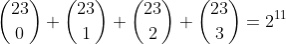Tweets about algebra, number theory, and miscellaneous math by @JohnDCook.
Nov 25 6 tweets 2 min read
You can use the quadratic formula without understanding the problem that lead to using it.

But the formula gives two roots, and you have to know which one makes sense in context. Now you do need to understand the application.

This is a simple example of a common pattern. A sighting of the sun or of a planet at a particular time determines a circle of possible locations.

A second sighting at a different time gives a second circle.

These two circles generally intersect in two points, one of which hopefully you know cannot be your location.
Feb 9 7 tweets 2 min read
Necessary conditions for a number ending in yz to be a square.

z must be 0, 1, 4, 5, 6, or 9.

If z = 1, 4, or 9, y must be even.

If z = 6, y must be odd.

If z = 0, y must be 0.

If z = 5, y must be 2. For example, can 2194 be a square? No, because 9 is odd.

Can 2184 be a square? Possibly, because 8 is even.

(In fact it's not a square. These are necessary conditions, but not sufficient.)
Dec 23, 2021 10 tweets 2 min readThe observation below led Marcel Golay to discover what’s now called the Golay code.The code uses blocks of 23 bits, 12 for data and 11 added for error detection.

It can correct up to 3 erroneous bits per block.
Jan 28, 2021 10 tweets 2 min read
How to mentally compute the cube root of the cube of a two digit number.

Thread (1/10) In base 10, the last digits of the cubes of digits are distinct.

If d = 0, 1, 4, 5, 6, or 9, then d^3 ends in d.

If d = 2, 3, 7, or 8, then d^3 ends in 10-d.

(2/10)